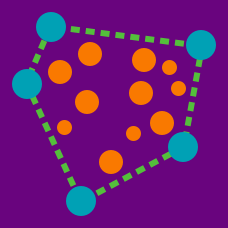Computer Science

# Intersections, Line Segments

Given that $P$ denotes a set of point, the pseudocode shown below checks if lines are collinear by checking if the point $p$,$q$, and $r$ are collinear, where $q$ is the central point, by checking if the the slopes of the segments $\overline { pq }$ and $\overline { qr }$ are identical.

  1 2 3 4 5 6 7 8 9 10 11 12 13 14 15 16 17 18 19 20 21 22 23 24 25 26 Function Check-For-Collinear(P,n) if (n ≤ 2) then return(false) else Sort the points in P based on their x-coordinates. for ( i = 1 to n) do Compute the slopes of the segments (P ¯ [i], P[j]) for each j = 1, 2,i − 1, i + 1, i + 2, . . . , n and store these in an auxiliary array A[i]. end for for ( i = 1 to n) do Sort the auxiliary array A[i]. end for for (i = 1 to n − 2) do for (j = i + 1 to n − 1) do Let x = slope(P ¯ [i], P[j]). Perform a binary search on A[j] to check if there is a point P[k] such that slope(P[j], P[k]) = x. if (such a point P[k] exists) then Points P[i], P[j] and P[k] are collinear. end if end for end for Declare that no three points are collinear. end if 

What is the worst case running time of the algorithm?

The following text file contains a set of triplets. Each triplets contains three integers $x,y,R$, representing a circle of radius $R$ centered at $(x,y)$. Out of each pair of circles in the file, how may of them intersect?

How many of the pairs of line segments in this text file intersect?

Details and assumption

• The text file contains 499 lists of integers in the following format $[a,b,c,d,e,f,g,h]$ this represents a set of four points representing two lines $AB$ and $CD$. Line segment $AB$ the coordinate $A=(a,b),B=(c,d)$ and line $CD$ is $C=(e,f),D=(g,h)$
×